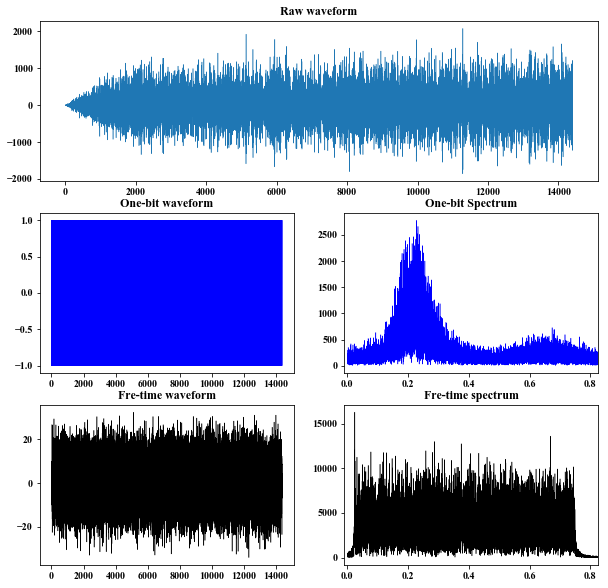New responses between station pairs can be extracted from diffuse wave field by cross-correlating ambient seismic noise or coda (Campillo & Paul, 2003; Bensen et al., 2007). However, some processing techniques should be taken into consideration to retrieve clear emperical green’s functions (EGFs). Here we show these steps in detail.

$\boxed{\text{Data processing steps}}$

### 5. Temperal normalization

$\boxed{\text{One-bit}}$ $$\tilde{d_j}=sign(d_j) \tag{1}$$ $\boxed{\text{run absolute mean}}$ \begin{aligned} w_j &= \frac{1}{2N+1}\sum_{n=j-N}^{j+N}|d_n| \\ \tilde{d_j} &= \frac{d_j}{w_j} \end{aligned} \tag{2}.

### 6. Spectral whitening

Using $\text{run absolute mean}$ algorithm. \begin{aligned} w_j &= \frac{1}{2N+1}|fd_j| \\ \tilde{fd_j} &= \frac{fd_j}{w_j} \end{aligned} \tag{3}.

Combining steps $5$ and $6$, an improved algrithm suggested by Shen et al. (2012) is given by $$y(t) = \sum_{f_k=f_1}^{f_2}\frac{x_{f_k, f_k+\Delta f}(t)}{Env[x_{f_k,f_k+\Delta f}(t)]} \tag{4},$$ where, in general, $\Delta f = \frac{f_1}{4}$ and $Env[x_{f_k, f_k+\Delta f}]$ is the envelope of $x_{f_k, f_k+\Delta f}(t)$.

  1 2 3 4 5 6 7 8 9 10 11 12 13 14 15 16 17 18 19 20 21 22 23 24 25 26 27 28 29 30 31 32 33 34 35 36 37 38 39 40 41 42 43 44 45 46 47 48 49 50 51 52 53 54 55 56 57 58 59 60 61 62 63 64 65 66 67 68 69 70 71 72 73 74 75  import numpy as np from scipy.signal import butter, filtfilt from obspy import read import matplotlib.pyplot as plt from scipy import fft def envelope(d): fd = fft.fft(d) n = len(fd) fd[1:n//2] *= 2. fd[n//2:] = 0. return (d**2+fft.ifft(fd).imag**2)**.5 def FTN1(tr, f1, f2, num=4): d = np.zeros_like(tr.data) df = f1 / num fl = f1 fh = fl + df while fh <= f2+df: trc = tr.copy() trc.filter('bandpass', freqmin=fl, freqmax=fh, corners=4, zerophase=True) d += (trc.data / envelope(trc.data)) fl += df; fh += df return d/(f2-f1)*df def FTN2(d1, fs, f1, f2, num=4.): d2 = np.zeros_like(d1) df = f1 / num; fl = f1 while fl <= f2: fh = fl + df [b, a] = butter(N=4, Wn=[2*fl/fs, 2*fh/fs], btype='bandpass') dd = filtfilt(b, a, d1) d2 += (dd/envelope(dd)) fl += df return d2 / (f2-f1) def main(): tr = read('Band_0.01-0.49/2019-09-04/HOUR_00-04/CO.BIRD.00.HHZ.SAC') tr.data /= 1e6 # tr.data = tr.data[len(tr.data)//20: 2*len(tr.data)//20] f1 = 0.01; f2 = 0.75 dt = tr.stats.delta; fs = 1 / dt tr.filter('bandpass', freqmin=f1, freqmax=f2, corners=10, zerophase=True) ob = np.sign(tr.data) fob = fft.fft(ob) nd = FTN2(tr.data, 1/dt, f1, f2, num=1) # nd = FTN1(tr, f1, f2, num=1) fnd = fft.fft(nd) n = len(ob) t = np.arange(n) * dt f = np.arange(n) * fs / n plt.figure(figsize=(10, 10)) plt.subplot(311) plt.plot(t, tr.data, lw=.5) plt.title('Raw waveform') plt.subplot(323) plt.plot(t, ob, lw=.5, color='b') plt.title('One-bit waveform') plt.subplot(325) plt.plot(t, nd, lw=.5, color='k') plt.title('Fre-time waveform') plt.subplot(324) plt.plot(f, abs(fob), lw=.5, color='b') plt.title('One-bit Spectrum') plt.xlim(-f1, f2*1.1) plt.subplot(326) plt.plot(f, abs(fnd), lw=.5, color='k') plt.title('Fre-time spectrum') plt.xlim(-f1, f2*1.1) plt.show() if __name__ =='__main__': main()### 7. Computing cross-correlation time functions.

$$C_{12}(t) = \int_{-\infty}^{\infty}u_1(\tau+t)u_2(\tau)d\tau \tag{5}.$$ Stacking all cross-correlations to get EGFs, $$EGF(t) = \frac{1}{N}\sum_{n=1}^{N}C_n(t) \tag{6}.$$

### References

Bensen, G. D. , Ritzwoller, M. H. , Barmin, M. P. , Levshin, A. L. , Lin, F. , & Moschetti, M. P. , et al. (0). Processing seismic ambient noise data to obtain reliable broad‐band surface wave dispersion measurements. Geophysical Journal International(3), 3.

Campillo, M. , & Paul, A. . (2003). Long-range correlations in the diffuse seismic coda. ence, 299(5606), 547-549.

Shen, Y. , Ren, Y. , Gao, H. , & Savage, B. . (2012). An improved method to extract very-broadband empirical green’s functions from ambient seismic noise. Bulletin of the Ssmological Society of America, 102(4), 1872-1877.